# LFSR -Linear Feedback Shift Register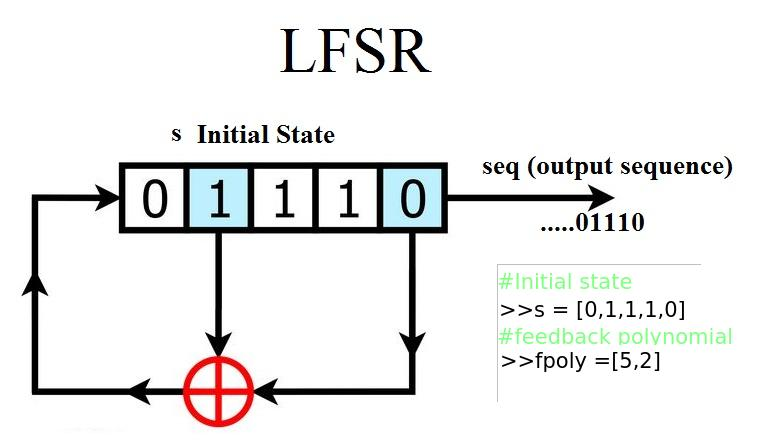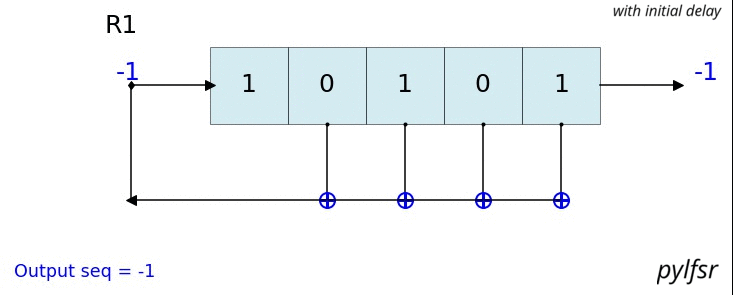## Plot Your LFSR with pylfsr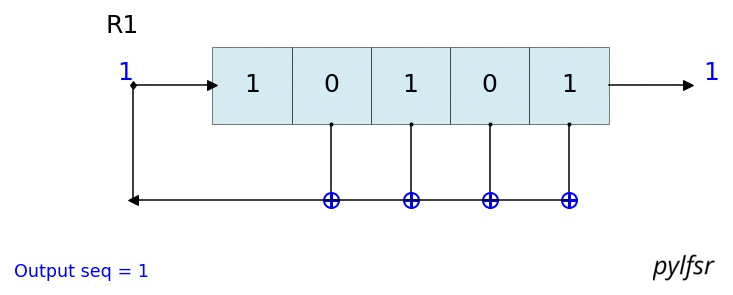• Fixed the bugs (1) missing initial bit (2) exception
• Added test properties of LFSR
• (1) Balance Property
• (2) Runlength Property
• (3) Autocorrelation Property
• Ploting function to display LFSR
• A5/1 GSM Stream Ciper Generator
• Geffe Generator

# Installation

## Requirement : numpy, matplotlib

### with pip

``````pip install pylfsr
``````

### Build from the source

Download the repository or clone it with git, after cd in directory build it from source with

``````python setup.py install
``````

## Examples

### Example 1: 5-bit LFSR with feedback polynomial x^5 + x^2 + 1

``````# import LFSR
import numpy as np
from pylfsr import LFSR

L = LFSR()

# print the info
L.info()

5 bit LFSR with feedback polynomial  x^5 + x^2 + 1
Expected Period (if polynomial is primitive) =  31
Current :
State        :  [1 1 1 1 1]
Count        :  0
Output bit   : -1
feedback bit : -1
``````
``````L.next()
L.runKCycle(10)
L.runFullCycle()
L.info()
``````

### Example 2**: 5-bit LFSR with custum state and feedback polynomial

``````state = [0,0,0,1,0]
fpoly = [5,4,3,2]
L = LFSR(fpoly=fpoly,initstate =state, verbose=True)
L.info()
tempseq = L.runKCycle(10)
L.set(fpoly=[5,3])
``````

### Example 3 ## To visualize the process with 3-bit LFSR, with default counter_start_zero = True

``````state = [1,1,1]
fpoly = [3,2]
L = LFSR(initstate=state,fpoly=fpoly)
print('count \t state \t\toutbit \t seq')
print('-'*50)
for _ in range(15):
print(L.count,L.state,'',L.outbit,L.seq,sep='\t')
L.next()
print('-'*50)
print('Output: ',L.seq)
``````

Output :

``````count 	        state 		outbit 	 seq
--------------------------------------------------
0		[1 1 1]		-1	[-1]
1		[0 1 1]		1	
2		[0 0 1]		1	[1 1]
3		[1 0 0]		1	[1 1 1]
4		[0 1 0]		0	[1 1 1 0]
5		[1 0 1]		0	[1 1 1 0 0]
6		[1 1 0]		1	[1 1 1 0 0 1]
7		[1 1 1]		0	[1 1 1 0 0 1 0]
8		[0 1 1]		1	[1 1 1 0 0 1 0 1]
9		[0 0 1]		1	[1 1 1 0 0 1 0 1 1]
10		[1 0 0]		1	[1 1 1 0 0 1 0 1 1 1]
11		[0 1 0]		0	[1 1 1 0 0 1 0 1 1 1 0]
12		[1 0 1]		0	[1 1 1 0 0 1 0 1 1 1 0 0]
13		[1 1 0]		1	[1 1 1 0 0 1 0 1 1 1 0 0 1]
14		[1 1 1]		0	[1 1 1 0 0 1 0 1 1 1 0 0 1 0]
--------------------------------------------------
Output:  [1 1 1 0 0 1 0 1 1 1 0 0 1 0 1]
``````

### Example 4 ## To visualize the process with 3-bit LFSR, with default counter_start_zero = False

``````state = [1,1,1]
fpoly = [3,2]
L = LFSR(initstate=state,fpoly=fpoly,counter_start_zero=False)
print('count \t state \t\toutbit \t seq')
print('-'*50)
for _ in range(15):
print(L.count,L.state,'',L.outbit,L.seq,sep='\t')
L.next()
print('-'*50)
print('Output: ',L.seq)
``````

Output

``````count 	 state 		outbit 	 seq
--------------------------------------------------
1	[1 1 1]		1	
2	[0 1 1]		1	[1 1]
3	[0 0 1]		1	[1 1 1]
4	[1 0 0]		0	[1 1 1 0]
5	[0 1 0]		0	[1 1 1 0 0]
6	[1 0 1]		1	[1 1 1 0 0 1]
7	[1 1 0]		0	[1 1 1 0 0 1 0]
8	[1 1 1]		1	[1 1 1 0 0 1 0 1]
9	[0 1 1]		1	[1 1 1 0 0 1 0 1 1]
10	[0 0 1]		1	[1 1 1 0 0 1 0 1 1 1]
11	[1 0 0]		0	[1 1 1 0 0 1 0 1 1 1 0]
12	[0 1 0]		0	[1 1 1 0 0 1 0 1 1 1 0 0]
13	[1 0 1]		1	[1 1 1 0 0 1 0 1 1 1 0 0 1]
14	[1 1 0]		0	[1 1 1 0 0 1 0 1 1 1 0 0 1 0]
--------------------------------------------------
Output:  [1 1 1 0 0 1 0 1 1 1 0 0 1 0 1]
``````

## Visualize & Plot LFSR

``````L.Viz(show=False, show_labels=False,title='R1')

``````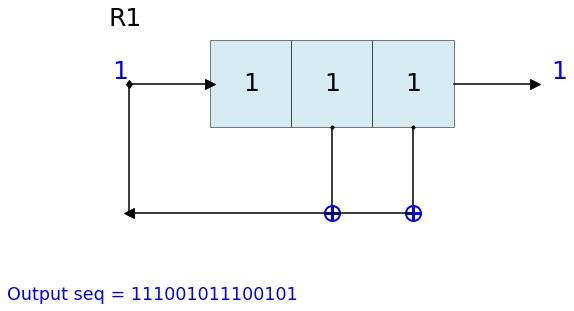### Dynamic plot - Animation in notebook

``````%matplotlib notebook
L = LFSR(initstate=[1,0,1,0,1],fpoly=[5,4,3,2],counter_start_zero=False)
fig, ax = plt.subplots(figsize=(8,3))
for _ in range(35):
ax.clear()
L.Viz(ax=ax, title='R1')
plt.ylim([-0.1,None])
#plt.tight_layout()
L.next()
fig.canvas.draw()
plt.pause(0.1)

``````## Example 5 ## 23 bit LFSR with custum state and feedback polynomial

``````fpoly = [23,19]
L1 = LFSR(fpoly=fpoly,initstate ='ones', verbose=False)
L1.info()
``````

Output

``````23 bit LFSR with feedback polynomial  x^23 + x^19 + 1
Expected Period (if polynomial is primitive) =  8388607
Current :
State        :  [1 1 1 1 1 1 1 1 1 1 1 1 1 1 1 1 1 1 1 1 1 1 1]
Count        :  0
Output bit   :  -1
feedback bit :  -1
``````
``````seq = L1.runKCycle(100)
``````
``````array([1, 1, 1, 1, 1, 1, 1, 1, 1, 1, 1, 1, 1, 1, 1, 1, 1, 1, 1, 1, 1, 1,
1, 0, 0, 0, 0, 0, 0, 0, 0, 0, 0, 0, 0, 0, 0, 0, 0, 0, 0, 0, 1, 1,
1, 1, 0, 0, 0, 0, 0, 0, 0, 0, 0, 0, 0, 0, 0, 0, 0, 1, 1, 1, 1, 1,
1, 1, 1, 0, 0, 0, 0, 0, 0, 0, 0, 0, 0, 0, 1, 1, 1, 1, 0, 0, 0, 0,
1, 1, 1, 1, 0, 0, 0, 0, 0, 0, 0, 1])
``````

## Example 6 ## testing the properties

``````state = [1,1,1,1,0]
fpoly = [5,3]
L = LFSR(initstate=state,fpoly=fpoly)
result  = L.test_properties(verbose=2)
``````

Output

``````1. Periodicity
------------------
- Expected period = 2^M-1 = 31
- Pass?:  True

2. Balance Property
-------------------
- Number of 1s = Number of 0s+1 (in a period): (N1s,N0s) =  (16, 15)
- Pass?:  True

3. Runlength Property
-------------------
- Number of Runs in a period should be of specific order, e.g. [4,2,1,1]
- Runs:  [8 4 2 1 1]
- Pass?:  True

4. Autocorrelation Property
-------------------
- Autocorrelation of a period should be noise-like, specifically, 1 at k=0, -1/m everywhere else
- Pass?:  True

==================
Passed all the tests
==================
``````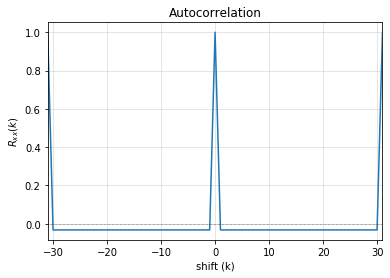Testing individual property

``````# get a full period sequence
p = L.getFullPeriod()
p
array([0, 1, 1, 1, 1, 1, 0, 0, 0, 1, 1, 0, 1, 1, 1, 0, 1, 0, 1, 0, 0, 0,
0, 1, 0, 0, 1, 0, 1, 1, 0])
``````
``````L.balance_property(p.copy())
# (True, (16, 15))

L.runlength_property(p.copy())
# (True, array([8, 4, 2, 1, 1]))

L.autocorr_property(p.copy())
#True
``````

## Example 7 ## testing the properties for non-primitive polynomial

``````state = [1,1,1,1,0]
fpoly = [5,1]
L = LFSR(initstate=state,fpoly=fpoly)
result = L.test_properties(verbose=1)
``````

Output

``````1. Periodicity
------------------
- Expected period = 2^M-1 = 31
- Pass?:  False

2. Balance Property
-------------------
- Number of 1s = Number of 0s+1 (in a period): (N1s,N0s) =  (17, 14)
- Pass?:  False

3. Runlength Property
-------------------
- Number of Runs in a period should be of specific order, e.g. [4,2,1,1]
- Runs:  [10  2  1  1  2]
- Pass?:  False

4. Autocorrelation Property
-------------------
- Autocorrelation of a period should be noise-like, specifically, 1 at k=0, -1/m everywhere else
- Pass?:  False

==================
Failed one or more tests, check if feedback polynomial is primitive polynomial
==================
``````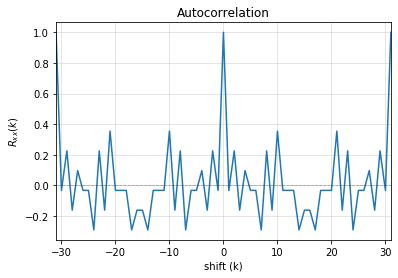### Example 8**: Get the feedback polynomial or list

Reference : http://www.partow.net/programming/polynomials/index.html

``````L = LFSR()
# list of 5-bit feedback polynomials
fpoly = L.get_fpolyList(m=5)

# list of all feedback polynomials as a dictionary
fpolyDict = L.get_fpolyList()
``````

### Changing feedback polynomial in between

``````L.changeFpoly(newfpoly =[23,14],reset=False)
seq1 = L.runKCycle(20)

# Change after 20 clocks
L.changeFpoly(newfpoly =[23,9],reset=False)
seq2 = L.runKCycle(20)
``````

# A5/1 GSM Stream cipher generatorRef: https://en.wikipedia.org/wiki/A5/1

``````import numpy as np
import matplotlib.pyplot as plt
from pylfsr import A5_1

A5 = A5_1(key='random')
print('key: ',A5.key)
A5.R1.Viz(title='R1')
A5.R2.Viz(title='R2')
A5.R3.Viz(title='R3')

print('key: ',A5.key)
print()
print('count \t cbit\t\tclk\t R1_R2_R3\toutbit \t seq')
print('-'*80)
for _ in range(15):
print(A5.count,A5.getCbits(),A5.clock_bit,A5.getLastbits(),A5.outbit,A5.getSeq(),sep='\t')
A5.next()
print('-'*80)
print('Output: ',A5.seq)

A5.runKCycle(1000)
A5.getSeq()

``````

## Enhanced A5/1

Reference Article: Enhancement of A5/1: https://doi.org/10.1109/ETNCC.2011.5958486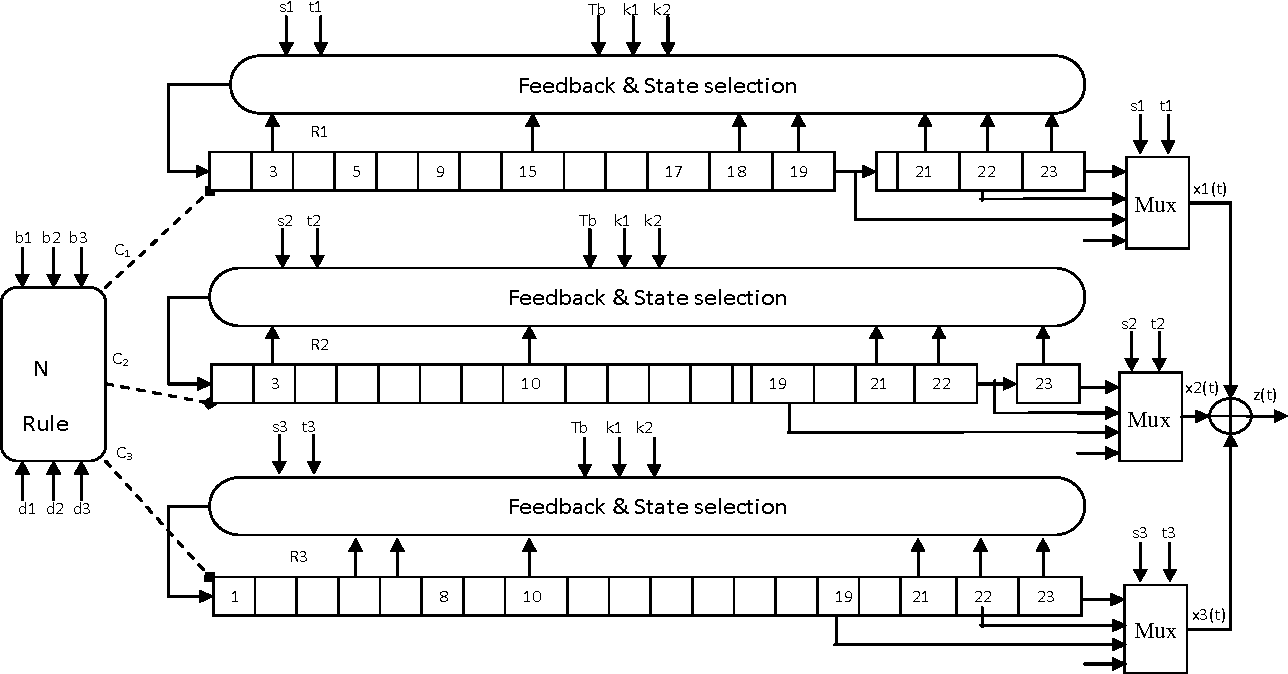``````# Three LFSRs initialzed with 'ones' though they are intialized with encription key
R1 = LFSR(fpoly = [19,18,17,14])
R2 = LFSR(fpoly = [23,22,21,8])
R3 = LFSR(fpoly = [22,21])

# clocking bits
b1 = R1.state
b2 = R3.state
b3 = R3.state

``````

# Geffe Generator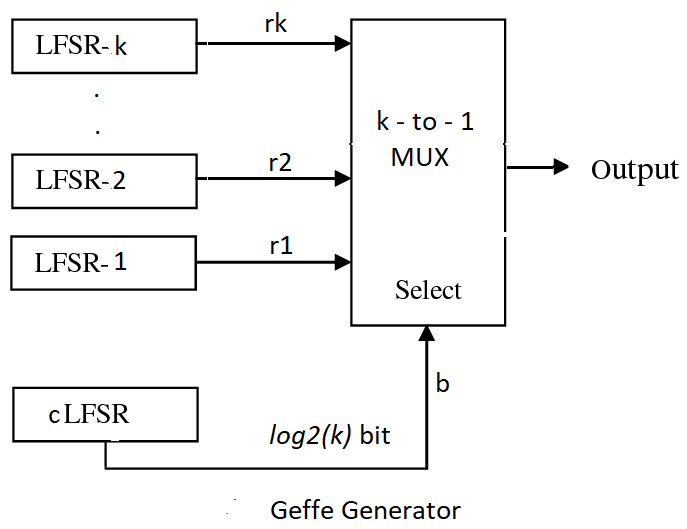Ref: Schneier, Bruce. Applied cryptography: protocols, algorithms, and source code in C. john wiley & sons, 2007. Chaper 16

``````import numpy as np
import matplotlib.pyplot as plt
from pylfsr import Geffe, LFSR

kLFSR = [LFSR(initstate='random') for _ in range(8)]  # List of 8 5-bit LFSRs with default feedback polynomial and random initial state
cLFSR = LFSR(initstate='random')                      # A 5-bit LFSR with for selecting one of 8 output at a time

GG = Geffe(kLFSR_list=kLFSR, cLFSR=cLFSR)

print('key: ',GG.getState())
print()
for _ in range(50):
print(GG.count,GG.m_count,GG.outbit_k,GG.sel_k,GG.outbit,GG.getSeq(),sep='\t')
GG.next()

GG.runKCycle(1000)
GG.getSeq()
``````

# MATLAB

Folder : https://github.com/Nikeshbajaj/Linear_Feedback_Shift_Register/tree/master/matlabfiles

Description Genrate randon binary sequence using LFSR for any given feedback taps (polynomial), This will also check Three fundamental Property of LFSR

1. Balance Property
2. Runlength Property
3. Autocorrelation Property

This MATLAB Code work for any length of LFSR with given taps (feedback polynomial) -Universal, There are three files LFSRv1.m an LFSRv2.m, LFSRv3.m

### LFSRv1

This function will return all the states of LFSR and will check Three fundamental Property of LFSR (1) Balance Property (2) Runlength Property (3) Autocorrelation Property

#### EXAMPLE

``````s=[1 1 0 0 1]
t=[5 2]
[seq c] =LFSRv1(s,t)
``````

### LFSRv2

This function will return only generated sequence will all the states of LFSR, no verification of properties are done here. Use this function to avoid verification each time you execute the program.

#### EXAMPLE

``````s=[1 1 0 0 1]
t=[5 2]
[seq c] =LFSRv2(s,t)
``````

### LFSRv3 (faster)

seq = LFSRv3(s,t,N) this function generates N bit sequence only. This is faster then other two functions, as this does not gives each state of LFSR

#### EXAMPLE

``````s=[1 1 0 0 1]
t=[5 2]
seq =LFSRv3(s,t,50)
``````

## Tips

• If you want to use this function in middle of any program, use LFSRv2 or LFSRv1 with verification =0.
• If you want to make it fast for long length of LFSR,use LFSRv3.m

# Cite As

``````@software{nikesh_bajaj_2021_4726667,
author       = {Nikesh Bajaj},
title        = {Nikeshbajaj/Linear\_Feedback\_Shift\_Register: 1.0.6},
month        = apr,
year         = 2021,
publisher    = {Zenodo},
version      = {1.0.6},
doi          = {10.5281/zenodo.4726667},
url          = {https://doi.org/10.5281/zenodo.4726667}
}
``````